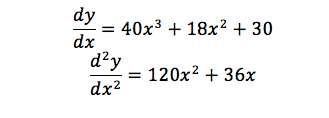top of page### Applications of Differentiation

#### Second Derivative

The second derivative is the derivative of the first derivate and is represented by the symbols below. In other words, it is the rate of change of the first derivative function.

Step 2: Differentiate the Equation Again

Make sure to include d^2y/dx^2 to show that it is the second derivative.

###### Worked Example of Second Derivative

In this example, we will differentiate y=(5x+3)(2x^3+6)

Step 1: Differentiate the equation.###### Applications of the Second Derivative

The second derivative is used for a variety of purposes, which will be explained in further detail in this section. Purposes include:

• Confirming whether a stationary point is a minimum, maximum or point of inflection through the second derivative test

• Using the second derivate to determine whether a stationary point is a minimum, maximum or point of inflection and using this information to sketch curves.

• Using the second derivative of displacement as a measure of acceleration

• Applying the second derivative to determine local minimum or maximum which can be applied to optimise the best outcome

bottom of page